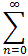#Interactive Real Analysis

Next | Previous | Glossary | Map

## 8.4. Taylor Series

### Example 8.4.5: A Taylor Series that does not Converge to its Function

Define. Show that:
• The function is infinitely often differentiable
• The Taylor series Tg(x, 0) around c = 0 has radius of convergence infinity.
• The Taylor series Tg(x, 0) around c = 0 does not converge to the original function.

We have already shown before that:

• g is infinitely often differentiable
• g(n)(0) = 0 for all n

The Taylor series of g is therefore:

Tg(x, 0) =an xn =1/n! g(n)(0) xn =0 xn = 0 for all x

In particular, the radius of convergence is infinity. But the original function is not identically equal to zero (g(0) = 0 but g(x) > 0 for x # 0), so that:

Tg(x, 0) # g(x) unless x = 0
Next | Previous | Glossary | Map